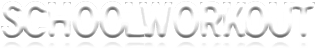A level Mathematics:  Resources for teachers

 MATHS PAGES FOR STUDENTS KS3 GCSE A LEVEL MATHS PAGES FOR TEACHERS KS3 RESOURCES GCSE RESOURCES A LEVEL RESOURCES HOME
 C1 AND C2 C3 AND C4 FURTHER PURE DECISION STATISTICS ASSESSMENTS

This web page contains handouts that can be photocopied and given to students to support A level mathematics teaching. It also contains end of unit assessments that can be used to assess student's learning (good for assessment for learning) as well as schemes of work.  All resources are written for the OCR A level mathematics specifications.

Core Mathematics C2

Handout on using integration to find areas (Word document)

Handout on the Quotient Rule (Word document)

Handout on Connected Rates of Change (Word document)

Handout on Decimal Search (Word document)

Core Mathematics C4

Handout on Binomial Expansions (Word document)

Handout on Implicit Differentiation (Word document)

Handout on Integration by Parts (Word document)

Handout on Differential Equations (Separating the Variables)  (Word document)

Handout on Vectors (Geometrical proof)  (Word document)

Handout on Vectors (position vectors, magnitude, direction, etc)  (Word document)

Modulus and argument of a complex number - multiplying and dividing complex numbers (Word document)

DeMoivre's Theorem - finding the nth root of a complex number (continued)  (Word document)

DeMoivre's Theorem - nth roots of unity (Word document)

Applications of DeMoivre's Theorem - expressing cos, sin and tan as powers of sinθ, cosθ and tanθ (Word document)

Applications of DeMoivre's Theorem - expressing powers of trigonometric ratios in terms of multiple angles (Word document)

Second order differential equations - finding a particular solution (Word document)

Cartesian and vector equation of a line in three dimensions  (Word document)

Equation of a plane - Cartesian and vector forms (Word document)

Subgroups - including Lagrange's Theorem  (Word document)

Isomorphic groups  (Word document)

Teaching notes on Graphs and Matrices (Word document)

Teaching notes on Prim's Algorithm (Word document)

Teaching notes on Bubble and Shuttle Sort (Word document)

Teaching notes on Bin Packing Algorithms (Word document)

Powerpoint presentation on networks.

Powerpoint presentation on the Simplex Algorithm.

Link to Mathsnet resources for Decision Maths (note that these are written for the Edexcel D1 syllabus).

Statistics 1 S1

Teaching notes on Standard Deviation from a Frequency Table (Word document)

Mean and standard deviation - coded data(Word document)

Coded data sorting activity  (Word document)

Introduction to Permutations (Word document)

Permutations with restrictions (Word document)

More permutations with restrictions(Word document)

Permutations and Combinations Card Sorting Activity (Word document)

Combinations (Word document)

Mean and variance of a Geometric Distribution(Word document)

Product Moment Correlation Coefficient (Word document)

Spearman's Rank Correlation Coefficient (Word document)

Statistics 2 S2

Teaching notes on Discrete Distributions (Word document)

What is the Distribution?  Sorting Activity (Word document)

Teaching notes on the Poisson Distribution (Word document)

Teaching notes on Approximating a Binomial by a Poisson Distribution (Word document)

Teaching notes on Approximating the Binomial and Poisson Distributions as a Normal (Word document)

Teaching notes on Sampling distributions (Exploring the Central Limit Theorem) (Word Document)

Teaching notes on Central Limit Theorem (Word document)

One-sided and Two-sided Hypothesis Tests using a Binomial Distribution (Powerpoint)

End of Topic Assessments and Schemes of Work

OCR Specification

 C1 and C2 C3 and C4 Further Pure C1 Scheme of Work Schedule Surds and powers Simultaneous equations Coordinate geometry Differentiation Further differentiation Transformation of graphs   C2 Scheme of Work Algebra and functions Logarithms Sequences and series (APs) Sequences and series (GPs) Integration Trigonometry Further trigonometry C3 Scheme of Work Schedule Functions Exponential functions Differentiation Integration Trigonometry Numerical methods     C4 Scheme of Work Schedule Algebra and functions Series and sequences Further differentiation Integration 1 Integration 2 Differential equations Vectors FP1 Schedule Introduction to matrices/ transformations Complex numbers 3 by 3 matrices and equations Loci (complex numbers) Series   FP3 Scheme of work Groups Further groups Complex numbers Differential equations Further differential equations

 Decision Maths Statistics Mechanics D1 Scheme of Work Algorithms Graph theory Prim's, Kruskal's and Dijstra's Simplex algorithm S1 Scheme of work Schedule Representing data (graphical) Measuring location and spread Probability Permutations/ combinations Discrete random variables Correlation Regression  S2 Scheme of work Schedule Hypothesis tests (discrete r.v.s) Type I/ II errors (discrete r.v.s) Sampling Hypothesis tests (normal mean) Type I/ II errors (normal) M2 Scheme of work